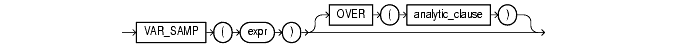Functions, 161 of 166

## VAR_SAMP

#### Syntax

var_samp::=Text description of `var_samp`

#### Purpose

`VAR_SAMP` returns the sample variance of a set of numbers after discarding the nulls in this set. You can use it as both an aggregate and analytic function.

The `expr` is a number expression, and the function returns a value of type `NUMBER`. If the function is applied to an empty set, it returns null. The function makes the following calculation:

```(SUM(expr2) - SUM(expr)2 / COUNT(expr)) / (COUNT(expr) - 1)

```

This function is similar to `VARIANCE`, except that given an input set of one element, `VARIANCE` returns 0 and `VAR_SAMP` returns null.

 See Also: "Aggregate Functions" "About SQL Expressions" for information on valid forms of `expr`

#### Aggregate Example

The following example returns the sample variance of the salaries in the sample `employees` table.

```SELECT VAR_SAMP(salary) FROM employees;

VAR_SAMP(SALARY)
----------------
15283140.5
```

#### Analytic Example

The following example calculates the cumulative population and sample variances of the monthly sales in 1998:

```SELECT t.calendar_month_desc,
VAR_POP(SUM(s.amount_sold))
OVER (ORDER BY t.calendar_month_desc) "Var_Pop",
VAR_SAMP(SUM(s.amount_sold))
OVER (ORDER BY t.calendar_month_desc) "Var_Samp"
FROM sales s, times t
WHERE s.time_id = t.time_id AND t.calendar_year = 1998
GROUP BY t.calendar_month_desc;

CALENDAR    Var_Pop   Var_Samp
-------- ---------- ----------
1998-01           0
1998-02  1.2844E+11 2.5687E+11
1998-03  9.1176E+10 1.3676E+11
1998-04  2.6891E+11 3.5855E+11
1998-05  1.9659E+12 2.4574E+12
1998-06  2.5810E+12 3.0972E+12
1998-07  3.5467E+12 4.1378E+12
1998-08  3.5100E+12 4.0114E+12
1998-09  3.3199E+12 3.7349E+12
1998-10  3.5001E+12 3.8890E+12
1998-11  3.2789E+12 3.6068E+12
1998-12  4.2486E+12 4.6348E+12
```